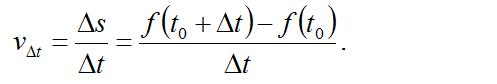# 导数的概念

1. 瞬时速度△s = f(t0 △t) – s0 = f(t0 △t)- f(t0)，

△s是物体在△t时间内移动的距离，是运动规律s = f(t ) 在时间 t0 。

p>

2. 切线斜率

y – f(x0) = k(x – x0)

3. 导数的概念

4. 示例

△y = f(x △x) – f(x) = sin(x △x) – sinx